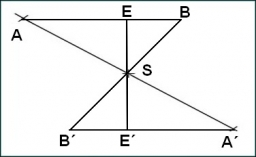# Determine 13541

The alpha angle is twice the beta angle, and the gamma angle is three times the beta angle. Determine the sizes of all interior angles of the alpha, beta, and gamma triangle ABC.

A =  60
B =  30
C =  90

### Step-by-step explanation:

A=2·B
C = 3·B
A+B+C=180

A-2B = 0
3B-C = 0
A+B+C = 180

Row 3 - Row 1 → Row 3
A-2B = 0
3B-C = 0
3B+C = 180

Row 3 - Row 2 → Row 3
A-2B = 0
3B-C = 0
2C = 180

C = 180/2 = 90
B = 0+C/3 = 0+90/3 = 30
A = 0+2B = 0+2 · 30 = 60

A = 60
B = 30
C = 90

Our linear equations calculator calculates it.Did you find an error or inaccuracy? Feel free to write us. Thank you!

Tips for related online calculators
Do you have a system of equations and looking for calculator system of linear equations?